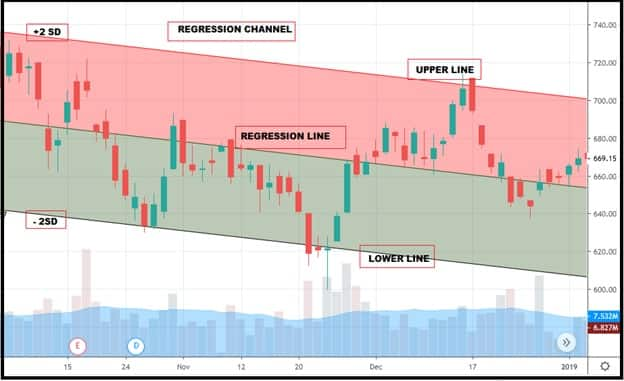# How to profitably trade using regression channel

## The Linear Regression indicator is typically used to analyze the upper and lower limits of an existing trend.

June 15, 2019 / 11:00 AM ISTShabbir Kayyumi

The indicator was developed by Gilbert Raff, and is sometimes referred to as the 'Raff Regression Channel'. The linear regression indicator is typically used to analyze the upper and lower limits of an existing trend.

What is a 'regression channel'?

Channels are an important component of technical analysis. There are quite a few types of channel trading techniques that can be applied. Some of these include Fibonacci channel, Andrews pitchfork, and the Keltner channel. The linear regression channel is a three-line technical indicator, which outlines the high, the low, and the middle of a trend or price move being analyzed.Figure .1.Illustration of regression channel

Construction of regression channel

Understanding underlying formula used for construction of a regression channel helps traders to take prudent decisions while trading complex scenarios.

Close
Calculating the indicator is no longer required, as charting platforms and trading software do it for us. Although knowing how the regression channel is calculated will help us better understand the indicator and its strengths and weaknesses.Figure .2. The regression channel construction

The regression channel is available on most trading platforms, such as Tradingview and MetaTrader. The indicator is also available on many free online charting sites, such as Investing.com, StockCharts.com and Yahoo! Finance.

Working of regression channel

- The width of the channel is based on the high or low that is the furthest from the linear regression.
-  There are two types of linear regression channel, depending on the direction of the trend – the bullish and the bearish linear regression channels. These two types of regression channels are defined based on the linear regression slope.
-  Technical and quantitative analysts have applied statistical principles to the financial market.
-  The bullish linear regression channel refers to bullish trends. In this case, the price is increasing and the slope of the linear regression is upwards.
-  The bearish linear regression channel is opposite to the bullish regression channel and it refers to bearish trends. For the bearish scenario, the price is decreasing and the slope of the regression channel is downward.Figure .3. The regression channel

- Like all technical indicators, it is important to use the regression channel in conjunction with other technical analysis tools.

The centerline is an important level to watch. Usage of Bollinger band and ADX is the most effective way while trading with a regression channel indicator. Bollinger band and a regression channel together are used to develop trading strategies. Key points about it are discussed below-Figure .4.Regression channel and signals

Entry
- Bollinger band: Bands of BB should be flat and the prices should touch the lower band.
- Regression channel lower line: When the prices are around the lower line of the regression channel.
- ADX: When the ADX indicator is trading below 20, it is an indication of consolidation or range bound movement.
Exit

- One can use multiple ways to book profits and exit, like prices near regression line or upper regression channel line.

Sell Signal:-
Entry
- Bollinger Band: Bands of BB should be flat and the prices should touch the upper band.
- Regression channel upper line: When price are around upper line of regression channel.

- ADX: When the ADX indicator is trading below 20, it is an indication of consolidation or range bound movement.

Exit

- One can use multiple ways to book profit and exit, like prices near regression line or lower regression channel line.

Conclusion
- Regression channel is a technical indicator that may offer potential buy and sell signals.
- The linear regression channel a.k.a. raff regression channel is a three line channel indicator based on standard deviation.
- When the trend is bullish and the indicator is upward sloping.

- When the trend is bearish and the indicator is downward sloping.

(The author is Head - Technical and Derivative Research at Narnolia Financial Advisors.)

Disclaimer: The views and investment tips expressed by investment expert on moneycontrol.com are his own and not that of the website or its management. Moneycontrol.com advises users to check with certified experts before taking any investment decisions.Moneycontrol Contributor
first published: Jun 15, 2019 10:59 am